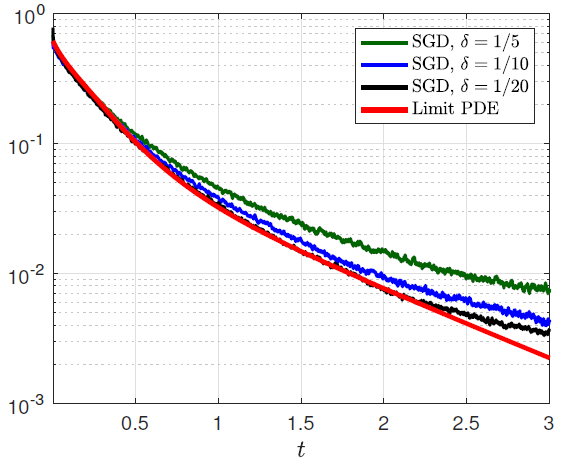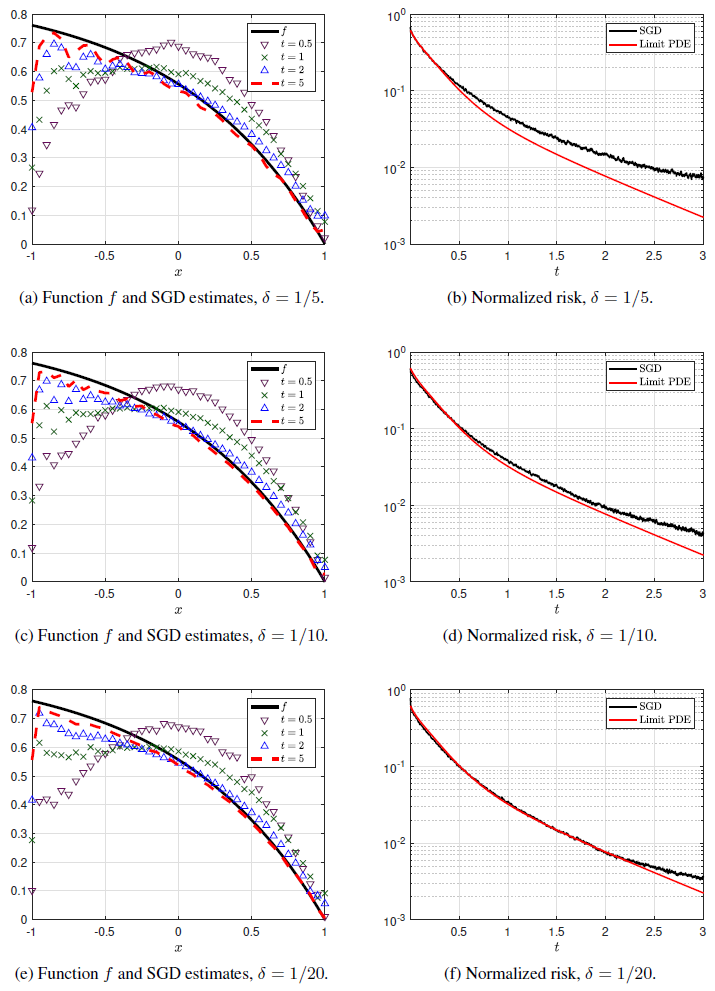## Abstract

We consider the problem of learning a function defined on a compact domain, using linear combinations of a large number of “bump-like” components (neurons). This idea lies at the core of a variety of methods from two-layer neural networks to kernel regression, to boosting. In general, the resulting risk minimization problem is non-convex and is solved by gradient descent or its variants. Nonetheless, little is known about global convergence properties of these approaches. In this work, we show that, as the number of neurons diverges and the bump width tends to zero, the gradient flow has a limit which is a viscous porous medium equation. By virtue of a property named “displacement convexity,” we show an exponential dimension-free convergence rate for gradient descent.This post is based on the paper .

Fitting a function with a linear combination of components. In supervised learning, we are given data points $$\{(y_j,{\boldsymbol{x}}_j)\}_{j\le n}$$, which are often assumed to be independent and identically distributed. Here $${\boldsymbol{x}}_j\in {\mathbb R}^d$$ is a feature vector, and $$y_j\in{\mathbb R}$$ is a label or response variable. We would like to fit a model $$\widehat{f}:{\mathbb R}^d\to{\mathbb R}$$ to predict the labels at new points $${\boldsymbol{x}}\in{\mathbb R}^d$$. One of the most fruitful ideas in this context is to use functions that are linear combinations of simple components: $\widehat{f}({\boldsymbol{x}};{\boldsymbol{w}}) = \frac{1}{N}\sum_{i=1}^N \sigma({\boldsymbol{x}};{\boldsymbol{w}}_i)\, ,$ where $$\sigma:{\mathbb R}^d\times{\mathbb R}^D\to{\mathbb R}$$ is a component function (a ‘neuron’ or ‘unit’ in the neural network parlance), and $${\boldsymbol{w}}=({\boldsymbol{w}}_1,\dots,{\boldsymbol{w}}_N)\in{\mathbb R}^{D\times N}$$ are the parameters to be learnt from data. Specific instantiations of this idea include, e.g., two-layer neural networks with radial activations, sparse deconvolution, kernel ridge regression, random feature methods, and boosting.

A common approach towards fitting parametric models is by risk minimization: $R_N({\boldsymbol{w}}) = {\mathbb E}\Big\{\Big[y-\frac{1}{N}\sum_{i=1}^N\sigma({\boldsymbol{x}};{\boldsymbol{w}}_i)\Big]^2\Big\}\, .$

Despite the impressive practical success of these methods, the risk function $$R_N({\boldsymbol{w}})$$ is highly non-convex and little is known about the global convergence of algorithms that try to minimize it. The main objective of this work is to introduce a nonparametric underlying regression model for which a global convergence result can be proved for stochastic gradient descent (SGD), in the limit of a large number of neurons.

Setting and main result. Let $$\Omega\subset{\mathbb R}^d$$ be a compact convex set with smooth boundary, and let $$\{(y_j,{\boldsymbol{x}}_j)\}_{j\ge 1}$$ be i.i.d. with $${\boldsymbol{x}}_j\sim {\sf Unif}(\Omega)$$ and $${\mathbb E}(y_j|{\boldsymbol{x}}_j)=f({\boldsymbol{x}}_j)$$, where the function $$f$$ is smooth. We try to fit these data using a combination of bumps, namely $\widehat{f}({\boldsymbol{x}};{\boldsymbol{w}})= \frac{1}{N}\sum_{i=1}^NK^\delta({\boldsymbol{x}}-{\boldsymbol{w}}_i)\, ,$ where $$K^\delta({\boldsymbol{x}}) = \delta^{-d}K({\boldsymbol{x}}/\delta)$$ and $$K:{\mathbb R}^d\to{\mathbb R}_{\ge 0}$$ is a first order kernel with compact support. The weights $${\boldsymbol{w}}_i\in{\mathbb R}^d$$ represent the centers of the bumps. Our model is general enough to include a broad class of radial-basis function (RBF) networks which are known to be universal function approximators. To the best of our knowledge, there is no result on the global convergence of stochastic gradient descent for learning RBF networks, and we establish the first result of this type.

We prove that, for sufficiently large $$N$$ and small $$\delta$$, gradient descent algorithms converge to weights $${\boldsymbol{w}}$$ with nearly optimum prediction error, provided $$f$$ is strongly concave. Let us emphasize that the resulting population risk $$R_N({\boldsymbol{w}})$$ is non-convex regardless of the concavity properties of $$f$$. Our proof unveils a novel mechanism by which global convergence takes place. Convergence results for non-convex empirical risk minimization are generally proved by carefully ruling out local minima in the cost function. Instead we prove that, as $$N\to\infty$$ and $$\delta\to 0$$, the gradient descent dynamics converges to a gradient flow in Wasserstein space, and that the corresponding cost function is ‘displacement convex.’ Breakthrough results in optimal transport theory guarantee dimension-free convergence rates for this limiting dynamics . In particular, we expect the cost function $$R_N({\boldsymbol{w}})$$ to have many local minima, which are however completely neglected by gradient descent.

Proof idea. Let us start by providing some high-level insights about our approach. Think about each model parameter as a particle moving under the effect of other particles according to the SGD updates. Now, instead of studying the microscopic dynamics of this system of particles, we analyze the macroscopic dynamics of the medium when the number of particles (i.e., the size of the hidden layer of the neural network) goes to infinity. These dynamics are formulated through a partial differential equation (more specifically, a viscous porous medium equation) that describes the evolution of the mass density over space and time. The nice feature of this approach is that, while the SGD trajectory is a random object, it shows that in the large particle size limit, it concentrates around the deterministic solution of this partial differential equation (PDE).

For a rigorous analysis and implementation of this idea, we use propagation-of-chaos techniques. Specifically, we show that, in the large $$N$$ limit, the evolution of the weights $$\{{\boldsymbol{w}}_i\}_{i=1}^N$$ under gradient descent can be replaced by the evolution of a probability distribution $$\rho^{\delta}_t$$ which satisfies the viscous porous medium PDE (with Neumann boundary conditions). This PDE can also be described as the Wasserstein $$W_2$$ gradient flow for the following effective risk $R^{\delta}(\rho) = \frac{1}{|\Omega|} \, \int_{\Omega} \big[f({\boldsymbol{x}}) - K^\delta\ast \rho({\boldsymbol{x}})\big]^2{\rm d}{\boldsymbol{x}}\, ,$ where $$|\Omega|$$ is the volume of the set $$\Omega$$ and $$\ast$$ is the usual convolution. The use of $$W_2$$ gradient flows to analyze two-layer neural networks was recently developed in several papers . However, we cannot rely on earlier results because of the specific boundary conditions in our problem.

Note that even though the cost $$R^{\delta}(\rho)$$ is quadratic and convex in $$\rho$$, its $$W_2$$ gradient flow can have multiple fixed points, and hence global convergence cannot be guaranteed. Indeed, the mathematical property that controls global convergence of $$W_2$$ gradient flows is not ordinary convexity but displacement convexity. Roughly speaking, displacement convexity is convexity along geodesics of the $$W_2$$ metric. Note that the risk function $$R^{\delta}(\rho)$$ is not even displacement convex. However, for small $$\delta$$, we can formally approximate $$K^\delta\ast \rho\approx \rho$$, and hence hope to replace the risk function $$R^{\delta}(\rho)$$ with the simpler one $R(\rho) = \frac{1}{|\Omega|}\int_{\Omega} \big[f({\boldsymbol{x}}) - \rho({\boldsymbol{x}})\big]^2{\rm d}{\boldsymbol{x}}\, .$ Most of our technical work is devoted to making rigorous this $$\delta\to 0$$ approximation.

Remarkably, the risk function $$R(\rho)$$ is strongly displacement convex (provided $$f$$ is strongly concave). A long line of work in PDE and optimal transport theory establishes dimension-free convergence rates for its $$W_2$$ gradient flow . By putting everything together, we are able to show that SGD converges exponentially fast to a near-global optimum with a rate that is controlled by the convexity parameter of $$f$$.

A numerical illustration. We demonstrate in a simple numerical example that the convergence rate predicted by our asymptotic theory is in excellent agreement with simulations. In the left column of the figure below, we plot the true function $$f$$ together with the neural network estimate obtained by running stochastic gradient descent (SGD) with $$N=200$$ neurons at several points in time $$t$$. Different plots correspond to different values of $$\delta$$ with $$\delta\in\{1/5, 1/10, 1/20\}$$. We observe that the network estimates converge to a limit curve which is an approximation of the true function $$f$$. As expected, the quality of the approximation improves as $$\delta$$ gets smaller. In the right column, we report the evolution of the population risk and we compare it to the risk predicted by the limit PDE (corresponding to $$\delta=0$$). The PDE curve appears to capture well the evolution of SGD towards optimality.## AuthorsAdel Javanmard, Data Science and Operations Department, Marshall School of Business, University of Southern CaliforniaMarco Mondelli, Institute of Science and Technology (IST) AustriaAndrea Montanari, Department of Electrical Engineering and Department of Statistics, Stanford University